# customblm

Bayesian linear regression model with custom joint prior distribution

## Description

The Bayesian linear regression model object customblm contains a log of the pdf of the joint prior distribution of (β,σ2). The log pdf is a custom function that you declare.

The data likelihood is $\prod _{t=1}^{T}\varphi \left({y}_{t};{x}_{t}\beta ,{\sigma }^{2}\right),$ where ϕ(yt;xtβ,σ2) is the Gaussian probability density evaluated at yt with mean xtβ and variance σ2. MATLAB® treats the prior distribution function as if it is unknown. Therefore, the resulting posterior distributions are not analytically tractable. To estimate or simulate from posterior distributions, MATLAB implements the slice sampler.

In general, when you create a Bayesian linear regression model object, it specifies the joint prior distribution and characteristics of the linear regression model only. That is, the model object is a template intended for further use. Specifically, to incorporate data into the model for posterior distribution analysis, pass the model object and data to the appropriate object function.

## Creation

### Syntax

PriorMdl = customblm(NumPredictors,'LogPDF',LogPDF)
PriorMdl = customblm(NumPredictors,'LogPDF',LogPDF,Name,Value)

### Description

example

PriorMdl = customblm(NumPredictors,'LogPDF',LogPDF) creates a Bayesian linear regression model object (PriorMdl) composed of NumPredictors predictors and an intercept, and sets the NumPredictors property. LogPDF is a function representing the log of the joint prior distribution of (β,σ2). PriorMdl is a template that defines the prior distributions and the dimensionality of β.

example

PriorMdl = customblm(NumPredictors,'LogPDF',LogPDF,Name,Value) sets properties (except NumPredictors) using name-value pair arguments. Enclose each property name in quotes. For example, customblm(2,'LogPDF',@logprior,'Intercept',false) specifies the function that represents the log of the joint prior density of (β,σ2), and specifies a regression model with 2 regression coefficients, but no intercept.

## Properties

expand all

You can set writable property values when you create the model object by using name-value pair argument syntax, or after you create the model object by using dot notation. For example, to exclude an intercept from the model, enter

PriorMdl.Intercept = false;

Number of predictor variables in the Bayesian multiple linear regression model, specified as a nonnegative integer.

NumPredictors must be the same as the number of columns in your predictor data, which you specify during model estimation or simulation.

When specifying NumPredictors, exclude any intercept term from the value.

After creating a model, if you change the value of NumPredictors using dot notation, then VarNames reverts to its default value.

Data Types: double

Flag for including a regression model intercept, specified as a value in this table.

ValueDescription
falseExclude an intercept from the regression model. Therefore, β is a p-dimensional vector, where p is the value of NumPredictors.
trueInclude an intercept in the regression model. Therefore, β is a (p + 1)-dimensional vector. This specification causes a T-by-1 vector of ones to be prepended to the predictor data during estimation and simulation.

If you include a column of ones in the predictor data for an intercept term, then set Intercept to false.

Example: 'Intercept',false

Data Types: logical

Predictor variable names for displays, specified as a string vector or cell vector of character vectors. VarNames must contain NumPredictors elements. VarNames(j) is the name of the variable in column j of the predictor data set, which you specify during estimation, simulation, or forecasting.

The default is {'Beta(1)','Beta(2),...,Beta(p)}, where p is the value of NumPredictors.

Example: 'VarNames',["UnemploymentRate"; "CPI"]

Data Types: string | cell | char

Log of the joint probability density function of (β,σ2), specified as a function handle in the form @fcnName, where fcnName is the function name.

Suppose logprior is the name of the MATLAB function defining the joint prior distribution of (β,σ2). Then, logprior must have this form.

function [logpdf,glpdf] = logprior(params) ... end
where:

• logpdf is a numeric scalar representing the log of the joint probability density of (β,σ2).

• glpdf is an (Intercept + NumPredictors + 1)-by-1 numeric vector representing the gradient of logpdf. Elements correspond to the elements of params.

glpdf is an optional output argument, and only the Hamiltonian Monte Carlo sampler (see hmcSampler) applies it. If you know the analytical partial derivative with respect to some parameters, but not others, then set the elements of glpdf corresponding to the unknown partial derivatives to NaN. MATLAB computes the numerical gradient for missing partial derivatives, which is convenient, but slows sampling.

• params is an (Intercept + NumPredictors + 1)-by-1 numeric vector. The first Intercept + NumPredictors elements must correspond to values of β, and the last element must correspond to the value of σ2. The first element of β is the intercept, if one exists. All other elements correspond to predictor variables in the predictor data, which you specify during estimation, simulation, or forecasting.

Example: 'LogPDF',@logprior

## Object Functions

 estimate Estimate posterior distribution of Bayesian linear regression model parameters simulate Simulate regression coefficients and disturbance variance of Bayesian linear regression model forecast Forecast responses of Bayesian linear regression model plot Visualize prior and posterior densities of Bayesian linear regression model parameters summarize Distribution summary statistics of standard Bayesian linear regression model

## Examples

collapse all

Consider the multiple linear regression model that predicts the US real gross national product (GNPR) using a linear combination of industrial production index (IPI), total employment (E), and real wages (WR).For alltime points,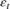is a series of independent Gaussian disturbances with a mean of 0 and variance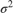. Assume these prior distributions:

•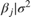is 4-D t distribution with 50 degrees of freedom for each component and the identity matrix for the correlation matrix. Also, the distribution is centered at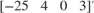and each component is scaled by the corresponding elements of the vector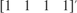.

•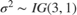.

bayeslm treats these assumptions and the data likelihood as if the corresponding posterior is analytically intractable.

Declare a MATLAB® function that:

• Accepts values of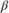andtogether in a column vector, and accepts values of the hyperparameters

• Returns the value of the joint prior distribution,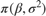, given the values ofandfunction logPDF = priorMVTIG(params,ct,st,dof,C,a,b) %priorMVTIG Log density of multivariate t times inverse gamma % priorMVTIG passes params(1:end-1) to the multivariate t density % function with dof degrees of freedom for each component and positive % definite correlation matrix C. priorMVTIG returns the log of the product of % the two evaluated densities. % % params: Parameter values at which the densities are evaluated, an % m-by-1 numeric vector. % % ct: Multivariate t distribution component centers, an (m-1)-by-1 % numeric vector. Elements correspond to the first m-1 elements % of params. % % st: Multivariate t distribution component scales, an (m-1)-by-1 % numeric (m-1)-by-1 numeric vector. Elements correspond to the % first m-1 elements of params. % % dof: Degrees of freedom for the multivariate t distribution, a % numeric scalar or (m-1)-by-1 numeric vector. priorMVTIG expands % scalars such that dof = dof*ones(m-1,1). Elements of dof % correspond to the elements of params(1:end-1). % % C: Correlation matrix for the multivariate t distribution, an % (m-1)-by-(m-1) symmetric, positive definite matrix. Rows and % columns correspond to the elements of params(1:end-1). % % a: Inverse gamma shape parameter, a positive numeric scalar. % % b: Inverse gamma scale parameter, a positive scalar. % beta = params(1:(end-1)); sigma2 = params(end); tVal = (beta - ct)./st; mvtDensity = mvtpdf(tVal,C,dof); igDensity = sigma2^(-a-1)*exp(-1/(sigma2*b))/(gamma(a)*b^a); logPDF = log(mvtDensity*igDensity); end 

Create an anonymous function that operates like priorMVTIG, but accepts only the parameter values, and holds the hyperparameter values fixed.

dof = 50; C = eye(4); ct = [-25; 4; 0; 3]; st = ones(4,1); a = 3; b = 1; logPDF = @(params)priorMVTIG(params,ct,st,dof,C,a,b); 

Create a custom joint prior model for the linear regression parameters. Specify the number of predictors p. Also, specify the function handle for priorMVTIG and the variable names.

p = 3; PriorMdl = bayeslm(p,'ModelType','custom','LogPDF',logPDF,... 'VarNames',["IPI" "E" "WR"]) 
PriorMdl = customblm with properties: NumPredictors: 3 Intercept: 1 VarNames: {4x1 cell} LogPDF: @(params)priorMVTIG(params,ct,st,dof,C,a,b) The priors are defined by the function: @(params)priorMVTIG(params,ct,st,dof,C,a,b) 

PriorMdl is a customblm Bayesian linear regression model object representing the prior distribution of the regression coefficients and disturbance variance. In this case, bayeslm does not display a summary of the prior distributions at the command line.

Consider the linear regression model in Create Custom Multivariate t Prior Model for Coefficients.

Create an anonymous function that operates like priorMVTIG, but accepts the parameter values only and holds the hyperparameter values fixed at their values.

dof = 50; C = eye(4); ct = [-25; 4; 0; 3]; st = ones(4,1); a = 3; b = 1; logPDF = @(params)priorMVTIG(params,ct,st,dof,C,a,b);

Create a custom joint prior model for the linear regression parameters. Specify the number of predictors p. Also, specify the function handle for priorMVTIG and the variable names.

p = 3; PriorMdl = bayeslm(p,'ModelType','custom','LogPDF',logPDF,... 'VarNames',["IPI" "E" "WR"])
PriorMdl = customblm with properties: NumPredictors: 3 Intercept: 1 VarNames: {4x1 cell} LogPDF: @(params)priorMVTIG(params,ct,st,dof,C,a,b) The priors are defined by the function: @(params)priorMVTIG(params,ct,st,dof,C,a,b) 

Load the Nelson-Plosser data set. Create variables for the response and predictor series.

load Data_NelsonPlosser X = DataTable{:,PriorMdl.VarNames(2:end)}; y = DataTable{:,'GNPR'};

Estimate the marginal posterior distributions of $\beta$ and ${\sigma }^{2}$. Specify a width for the slice sampler that is close to the posterior standard deviation of the parameters assuming a diffuse prior model. Reduce serial correlation by specifying a thinning factor of 10, and reduce the effective default number of draws by a factor of 10.

width = [20,0.5,0.01,1,20]; thin = 10; numDraws = 1e5/thin; rng(1) % For reproducibility PosteriorMdl = estimate(PriorMdl,X,y,'Width',width,'Thin',thin,... 'NumDraws',numDraws);
Method: MCMC sampling with 10000 draws Number of observations: 62 Number of predictors: 4 | Mean Std CI95 Positive Distribution -------------------------------------------------------------------------- Intercept | -25.0069 0.9919 [-26.990, -23.065] 0.000 Empirical IPI | 4.3544 0.1083 [ 4.143, 4.562] 1.000 Empirical E | 0.0011 0.0002 [ 0.001, 0.001] 1.000 Empirical WR | 2.5613 0.3293 [ 1.939, 3.222] 1.000 Empirical Sigma2 | 47.0593 8.7570 [32.690, 67.115] 1.000 Empirical 

PosteriorMdl is an empiricalblm model object storing draws from the posterior distributions of $\beta$ and ${\sigma }^{2}$ given the data. estimate displays a summary of the marginal posterior distributions to the command window. Rows of the summary correspond to regression coefficients and the disturbance variance, and columns to characteristics of the posterior distribution. The characteristics include:

• CI95, which contains the 95% Bayesian equitailed credible intervals for the parameters. For example, the posterior probability that the regression coefficient of WR is in [1.939, 3.222] is 0.95.

• Positive, which contains the posterior probability that the parameter is greater than 0. For example, the probability that the intercept is greater than 0 is 0.

estimate derives the posterior characteristics from draws from the posterior distributions, which MATLAB® stores as matrices in the properties BetaDraws and Sigma2Draws.

To monitor mixing and convergence of the MCMC sample, construct trace plots. In the BetaDraws property, draws correspond to columns and parameters to rows.

figure; for j = 1:4 subplot(2,2,j) plot(PosteriorMdl.BetaDraws(j,:)) title(sprintf('Trace Plot of %s',PosteriorMdl.VarNames{j})); end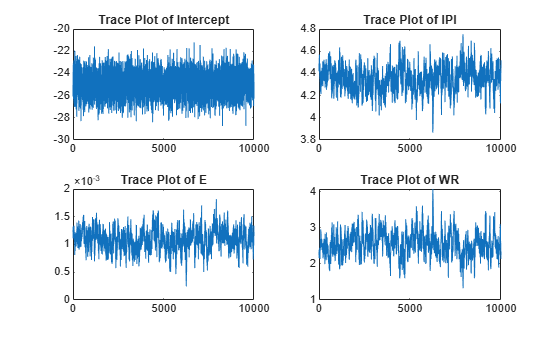figure; plot(PosteriorMdl.Sigma2Draws) title('Trace Plot of Sigma2');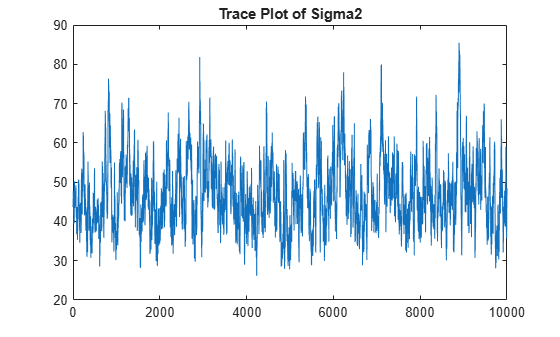The trace plots indicate adequate mixing and convergence, and there are no transient effects to remove.

Consider the linear regression model in Create Custom Multivariate t Prior Model for Coefficients.

Create an anonymous function that operates like priorMVTIG, but accepts the parameter values only and holds the hyperparameter values fixed.

dof = 50; C = eye(4); ct = [-25; 4; 0; 3]; st = ones(4,1); a = 3; b = 1; logPDF = @(params)priorMVTIG(params,ct,st,dof,C,a,b);

Create a custom joint prior model for the linear regression parameters. Specify the number of predictors p. Also, specify the function handle for priorMVTIG and the variable names.

p = 3; PriorMdl = bayeslm(p,'ModelType','custom','LogPDF',logPDF,... 'VarNames',["IPI" "E" "WR"])
PriorMdl = customblm with properties: NumPredictors: 3 Intercept: 1 VarNames: {4x1 cell} LogPDF: @(params)priorMVTIG(params,ct,st,dof,C,a,b) The priors are defined by the function: @(params)priorMVTIG(params,ct,st,dof,C,a,b) 

Load the Nelson-Plosser data set. Create variables for the response and predictor series.

load Data_NelsonPlosser X = DataTable{:,PriorMdl.VarNames(2:end)}; y = DataTable{:,'GNPR'};

Estimate the conditional posterior distribution of $\beta$ given the data and ${\sigma }^{2}=2$, and return the estimation summary table to access the estimates. Specify a width for the slice sampler that is close to the posterior standard deviation of the parameters assuming a diffuse prior model. Reduce serial correlation by specifying a thinning factor of 10, and reduce the effective default number of draws by a factor of 10.

width = [20,0.5,0.01,1]; thin = 10; numDraws = 1e5/thin; rng(1) % For reproducibility [Mdl,Summary] = estimate(PriorMdl,X,y,'Sigma2',2,... 'Width',width,'Thin',thin,'NumDraws',numDraws);
Method: MCMC sampling with 10000 draws Conditional variable: Sigma2 fixed at 2 Number of observations: 62 Number of predictors: 4 | Mean Std CI95 Positive Distribution -------------------------------------------------------------------------- Intercept | -24.7820 0.8767 [-26.483, -23.054] 0.000 Empirical IPI | 4.3825 0.0254 [ 4.332, 4.431] 1.000 Empirical E | 0.0011 0.0000 [ 0.001, 0.001] 1.000 Empirical WR | 2.4752 0.0724 [ 2.337, 2.618] 1.000 Empirical Sigma2 | 2 0 [ 2.000, 2.000] 1.000 Empirical 

estimate displays a summary of the conditional posterior distribution of $\beta$. Because ${\sigma }^{2}$ is fixed at 2 during estimation, inferences on it are trivial.

Extract the mean vector and covariance matrix of the conditional posterior of $\beta$ from the estimation summary table.

condPostMeanBeta = Summary.Mean(1:(end - 1))
condPostMeanBeta = 4×1 -24.7820 4.3825 0.0011 2.4752 
CondPostCovBeta = Summary.Covariances(1:(end - 1),1:(end - 1))
CondPostCovBeta = 4×4 0.7686 0.0084 -0.0000 0.0019 0.0084 0.0006 0.0000 -0.0015 -0.0000 0.0000 0.0000 -0.0000 0.0019 -0.0015 -0.0000 0.0052 

Display Mdl.

Mdl
Mdl = customblm with properties: NumPredictors: 3 Intercept: 1 VarNames: {4x1 cell} LogPDF: @(params)priorMVTIG(params,ct,st,dof,C,a,b) The priors are defined by the function: @(params)priorMVTIG(params,ct,st,dof,C,a,b) 

Because estimate computes the conditional posterior distribution, it returns the original prior model, not the posterior, in the first position of the output argument list. Also, estimate does not return the MCMC sample. Therefore, to monitor convergence of the MCMC sample, use simulate instead and specify the same random number seed.

Consider the linear regression model in Estimate Marginal Posterior Distributions.

Create a prior model for the regression coefficients and disturbance variance, then estimate the marginal posterior distributions. Turn the estimation display off.

dof = 50; C = eye(4); ct = [-25; 4; 0; 3]; st = ones(4,1); a = 3; b = 1; logPDF = @(params)priorMVTIG(params,ct,st,dof,C,a,b); p = 3; PriorMdl = bayeslm(p,'ModelType','custom','LogPDF',logPDF,... 'VarNames',["IPI" "E" "WR"]); load Data_NelsonPlosser X = DataTable{:,PriorMdl.VarNames(2:end)}; y = DataTable{:,'GNPR'}; width = [20,0.5,0.01,1,20]; thin = 10; numDraws = 1e5/thin; rng(1) % For reproducibility PosteriorMdl = estimate(PriorMdl,X,y,'Width',width,'Thin',thin,... 'NumDraws',numDraws,'Display',false);

Estimate posterior distribution summary statistics for $\beta$ by using the draws from the posterior distribution stored in posterior model.

estBeta = mean(PosteriorMdl.BetaDraws,2); EstBetaCov = cov(PosteriorMdl.BetaDraws');

Suppose that if the coefficient of real wages (WR)is below 2.5, then a policy is enacted. Although the posterior distribution of WR is known, and you can calculate probabilities directly, you can estimate the probability using Monte Carlo simulation instead.

Draw 1e6 samples from the marginal posterior distribution of $\beta$.

NumDraws = 1e6; BetaSim = simulate(PosteriorMdl,'NumDraws',NumDraws);

BetaSim is a 4-by- 1e6 matrix containing the draws. Rows correspond to the regression coefficient and columns to successive draws.

Isolate the draws corresponding to the coefficient of WR, and then identify which draws are less than 2.5.

isWR = PosteriorMdl.VarNames == "WR"; wrSim = BetaSim(isWR,:); isWRLT2p5 = wrSim < 2.5;

Find the marginal posterior probability that the regression coefficient of WR is below 2.5 by computing the proportion of draws that are less than 2.5.

probWRLT2p5 = mean(isWRLT2p5)
probWRLT2p5 = 0.4430 

The posterior probability that the coefficient of WR is less than 2.5 is about 0.4430.

Consider the linear regression model in Estimate Marginal Posterior Distributions.

Create a prior model for the regression coefficients and disturbance variance, then estimate the marginal posterior distributions. Hold out the last 10 periods of data from estimation so you can use them to forecast real GNP. Turn the estimation display off.

load Data_NelsonPlosser VarNames = {'IPI'; 'E'; 'WR'}; fhs = 10; % Forecast horizon size X = DataTable{1:(end - fhs),VarNames}; y = DataTable{1:(end - fhs),'GNPR'}; XF = DataTable{(end - fhs + 1):end,VarNames}; % Future predictor data yFT = DataTable{(end - fhs + 1):end,'GNPR'}; % True future responses dof = 50; C = eye(4); ct = [-25; 4; 0; 3]; st = ones(4,1); a = 3; b = 1; logPDF = @(params)priorMVTIG(params,ct,st,dof,C,a,b); p = 3; PriorMdl = bayeslm(p,'ModelType','custom','LogPDF',logPDF,... 'VarNames',VarNames); width = [20,0.5,0.01,1,20]; thin = 10; numDraws = 1e5/thin; rng(1) % For reproducibility PosteriorMdl = estimate(PriorMdl,X,y,'Width',width,'Thin',thin,... 'NumDraws',numDraws,'Display',false);

Forecast responses using the posterior predictive distribution and the future predictor data XF. Plot the true values of the response and the forecasted values.

yF = forecast(PosteriorMdl,XF); figure; plot(dates,DataTable.GNPR); hold on plot(dates((end - fhs + 1):end),yF) h = gca; hp = patch([dates(end - fhs + 1) dates(end) dates(end) dates(end - fhs + 1)],... h.YLim([1,1,2,2]),[0.8 0.8 0.8]); uistack(hp,'bottom'); legend('Forecast Horizon','True GNPR','Forecasted GNPR','Location','NW') title('Real Gross National Product'); ylabel('rGNP'); xlabel('Year'); hold off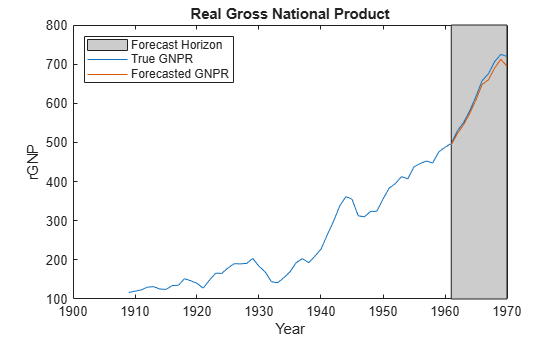yF is a 10-by-1 vector of future values of real GNP corresponding to the future predictor data.

Estimate the forecast root mean squared error (RMSE).

frmse = sqrt(mean((yF - yFT).^2))
frmse = 12.8148 

The forecast RMSE is a relative measure of forecast accuracy. Specifically, you estimate several models using different assumptions. The model with the lowest forecast RMSE is the best-performing model of the ones being compared.

expand all

## Alternatives

The bayeslm function can create any supported prior model object for Bayesian linear regression.

## Version History

Introduced in R2017a